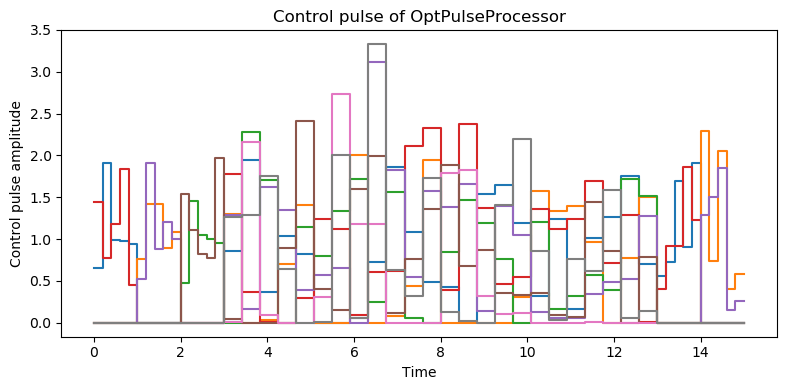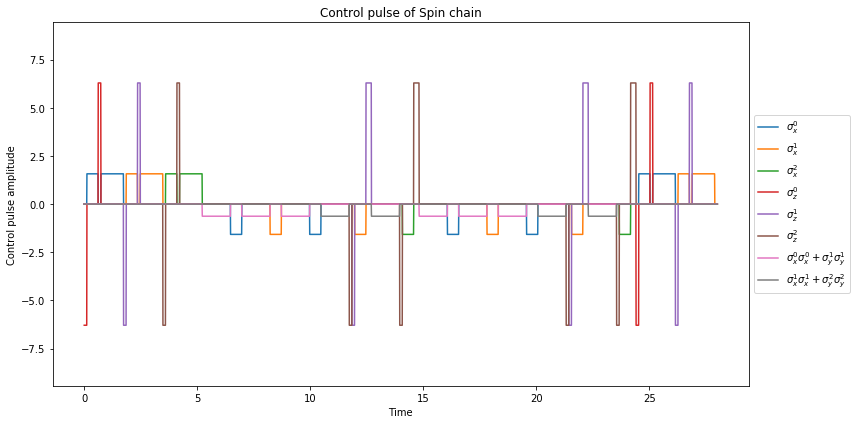# Running the Deutsch–Jozsa algorithm on the noisy device simulator¶

Author: Boxi Li ([email protected])

In this example, we demonstrate how to simulate simple quantum algorithms on a qauntum hardware with QuTiP. The simulators are defined in the class Processor(and its sub-classes). Processor represents a general quantum device. The interaction of the quantum systems such as qubits is defined by the control Hamiltonian. One can set the amplitude of the interaction by the attribute coeff which corresponds to the pulse intensity of the control system. For more details please refer to the introductory notebook.

In this example, we won't set the interaction strength by ourselves. Instead, we give it a sequence of gates, i.e. a QubitCircuit, and let the Processor find the desired pulses. The Processor class has a method load_circuit that can transfer a QubitCircuit object into a control pulse sequence. Different sub-class of Processor find their pulses in different ways. We show two examples here, one is based on a physical model and the other uses the qutip.control module. For each case, we also compare the result with or without noise by defining the t1 and t2 time of the device.

## The Deutsch–Jozsa algorithm¶

The Deutsch–Jozsa algorithm is the simplest quantum algorithm that offers an exponential speed-up compared to the classical one. It assumes that we have a function $f:\{0,1\}^n \rightarrow \{0,1\}$ which is either balanced or constant. Constant means that $f(x)$ is either 1 or 0 for all inputs while balanced means that $f(x)$ is 1 for half of the input domain and 0 for the other half. A more rigorous definition can be found at https://en.wikipedia.org/wiki/Deutsch-Jozsa_algorithm.

The implementation of the Deutsch–Jozsa algorithm inclues $n$ input qubits and 1 ancilla initialised in state $1$. At the end of the algorithm, the first $n$ qubits are measured on the computational basis. If the function is constant, the result will be $0$ for all $n$ qubits. If balanced, $\left|00...0\right\rangle$ will never be measured. The following example is implemented for the balanced function $f:\{00,01,10,11\} \rightarrow \{0,1\}$, where $f(00)=f(11)=0$ and $f(01)=f(10)=1$. This function is balanced, so the probability of measuring state $\left|00\right\rangle$ should be 0.

In :
import numpy as np
from qutip.qip.device import OptPulseProcessor, LinearSpinChain
from qutip.qip.circuit import QubitCircuit
from qutip.operators import sigmaz, sigmax, identity
from qutip.tensor import tensor
from qutip.states import basis
from qutip.qobj import ptrace
basis00 = tensor([basis(2,0), basis(2,0)])

In :
qc = QubitCircuit(N=3)

# function f(x)



## Using the optimal control module to find the pulse¶

This feature integrated into the sub-class OptPulseProcessor which use methods in the optimal control module to find the optimal pulse sequence for the desired gates. It can find the optimal pulse either for the whole unitary evolution or for each gate. Here we choose the second option.

In :
setting_args = {"SNOT": {"num_tslots": 5, "evo_time": 1},
"CNOT": {"num_tslots": 12, "evo_time": 5}}
processor = OptPulseProcessor(N=3)
amp_ubound=5, amp_lbound=0);

********** Gate 0 **********
Final fidelity error 1.9392365491199826e-10
Terminated due to function converged
Number of iterations 15
********** Gate 1 **********
Final fidelity error 6.092241155997158e-10
Terminated due to function converged
Number of iterations 16
********** Gate 2 **********
Final fidelity error 4.0477787788262276e-10
Terminated due to function converged
Number of iterations 19
********** Gate 3 **********
Final fidelity error 1.254523354754511e-06
Terminated due to function converged
Number of iterations 139
********** Gate 4 **********
Final fidelity error 2.349601027262782e-05
Terminated due to function converged
Number of iterations 36
********** Gate 5 **********
Final fidelity error 2.9691660241581985e-10
Terminated due to function converged
Number of iterations 16
********** Gate 6 **********
Final fidelity error 2.81108802901997e-10
Terminated due to function converged
Number of iterations 16


To quickly visualize the pulse, Processor has a method called plot_pulses. In the figure bellow, each colour represents the pulse sequence of one control Hamiltonian in the system as a function of time. In each time interval, the pulse remains constant.

In :
processor.plot_pulses(title="Control pulse of OptPulseProcessor", figsize=(8, 4), dpi=100);To simulate the evolution, we only need to call the method run_state which calls one of the open system solvers in QuTiP and calculate the time evolution.

### Without decoherence¶

In :
psi0 = tensor([basis(2, 0), basis(2, 0), basis(2, 1)])
result = processor.run_state(init_state=psi0)
print("Probability of measuring state 00:")
print(np.real((basis00.dag() * ptrace(result.states[-1], [0,1]) * basis00)[0,0]))

Probability of measuring state 00:
7.686362456299262e-07


### With decoherence¶

In :
processor.t1 = 100
processor.t2 = 30
psi0 = tensor([basis(2, 0), basis(2, 0), basis(2, 1)])
result = processor.run_state(init_state=psi0)
print("Probability of measuring state 00:")
print(np.real((basis00.dag() * ptrace(result.states[-1], [0,1]) * basis00)[0,0]))

Probability of measuring state 00:
0.09532818184024891


We can see that under noisy evolution their is a none zero probability of measuring state 00.

## Generating pulse based on quantum computing model¶

Below, we simulate the same quantum circuit using one sub-class LinearSpinChain. It will find the pulse based on the Hamiltonian available on a quantum computer of the linear spin chain system. Please refer to the notebook of the spin chain model for more details.

In :
processor2 = LinearSpinChain(3)

In :
processor2.plot_pulses(title="Control pulse of Spin chain");The first three pulse periods (from $t=0$ to $t\approx5$) are for the three Hadamard gates, they are followed by two long periods for the CNOT gates and then again two Hadamard. Different colours represent different kinds of interaction, as shown in the legend.

### Without decoherence¶

In :
psi0 = tensor([basis(2, 0), basis(2, 0), basis(2, 1)])
result = processor2.run_state(init_state=psi0)
print("Probability of measuring state 00:")
print(np.real((basis00.dag() * ptrace(result.states[-1], [0,1]) * basis00)[0,0]))

Probability of measuring state 00:
1.8002112771931593e-09


### With decoherence¶

In :
processor2.t1 = 100
processor2.t2 = 30
psi0 = tensor([basis(2, 0), basis(2, 0), basis(2, 1)])
result = processor2.run_state(init_state=psi0)
print("Probability of measuring state 00:")
print(np.real((basis00.dag() * ptrace(result.states[-1], [0,1]) * basis00)[0,0]))

Probability of measuring state 00:
0.1661582845316164

In :
from qutip.ipynbtools import version_table
version_table()

Out:
SoftwareVersion